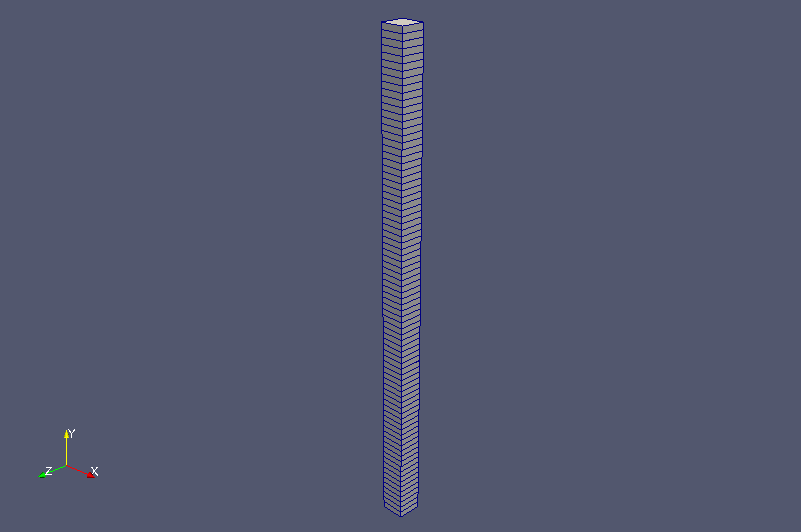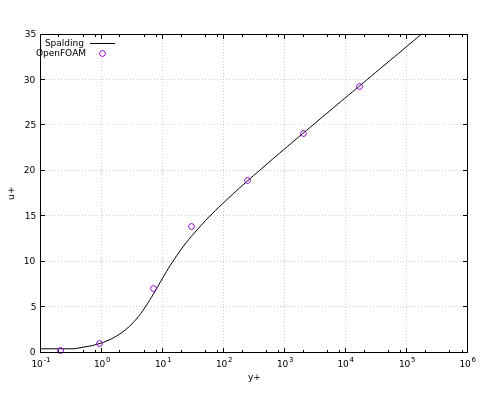﻿ Validation of wall function calculations - XSim

# Validation of wall function calculations

Update: June 1, 2017
OpenFOAM 4.x

$FOAM_TUTORIALS/incompressible/boundaryFoam/boundaryWallFunctionsProfile ## Summary We calculate the flow with the kinematic viscosity ν which is changed to 1e-2, 1e-3, 1e-4, 1e-5, 1e-6, 1e-7, and 1e-8 (in m2/s), respectively, and from the results, get the dimensionless distance and the dimensionless velocity from the cell adjacent to the wall. Then we compare them with the Spalding's law. The dimensionless distance y+, the dimensionless velocity u+, and the Spalding's law are defined as follows. • The dimensionless distance from wall:• the dimensionless velocity:• Spalding's law:where uτ is the wall friction velocity (= (wall shear stress/fluid density)1/2), y is the distance between the center of the cell adjacent to the wall and the wall, u is the flow velocity at the center of the cell adjacent to the wall, and ν is the kinematic viscosity. And, κ = 0.41 and E = 9.8 are used. Periodic boundary conditions are set for the inflow region "front" and the outflow region "back", and no-slip wall conditions are set for the channel wall regions "lowerWall" and "upperWall". The mesh is created only in the Y direction and the calculation is performed as a 2-dimensional model. The target mean velocity of 10 m/s for each kinematic viscosity is specified in the file constant/transportProperties as follows. Ubar [0 1 -1 0 0 0 0] (10 0 0);  The meshes are as follows.Meshes The calculation result is as follows.y+ vs. u+ (The circular marker is the calculated value and the line is the Spalding's law) The calculated values are approximately consistent with the Spalding's law. ## Commands cp -r$FOAM_TUTORIALS/incompressible/boundaryFoam/boundaryWallFunctionsProfile boundaryWallFunctionsProfile
cd boundaryWallFunctionsProfile

blockMesh
echo "# yPlus vs uPlus" > yPlus_vs_uPlus

# Calculation with nu = 1e-2
sed "s/XXX/2/g" constant/transportProperties.template > constant/transportProperties
boundaryFoam > log.boundaryFoam.2
foamLog log.boundaryFoam.2
yPlus=awk < logs/yPlus_0 'END{print $2}' uPlus=awk < logs/uPlus_0 'END{print$2}'
echo "$yPlus$uPlus" >> yPlus_vs_uPlus
rm -rf logs

# Calculation with nu = 1e-3
sed "s/XXX/3/g" constant/transportProperties.template > constant/transportProperties
boundaryFoam > log.boundaryFoam.3
foamLog log.boundaryFoam.3
yPlus=awk < logs/yPlus_0 'END{print $2}' uPlus=awk < logs/uPlus_0 'END{print$2}'
echo "$yPlus$uPlus" >> yPlus_vs_uPlus
rm -rf logs

# Calculation with nu = 1e-4
sed "s/XXX/4/g" constant/transportProperties.template > constant/transportProperties
boundaryFoam > log.boundaryFoam.4
foamLog log.boundaryFoam.4
yPlus=awk < logs/yPlus_0 'END{print $2}' uPlus=awk < logs/uPlus_0 'END{print$2}'
echo "$yPlus$uPlus" >> yPlus_vs_uPlus
rm -rf logs

# Calculation with nu = 1e-5
sed "s/XXX/5/g" constant/transportProperties.template > constant/transportProperties
boundaryFoam > log.boundaryFoam.5
foamLog log.boundaryFoam.5
yPlus=awk < logs/yPlus_0 'END{print $2}' uPlus=awk < logs/uPlus_0 'END{print$2}'
echo "$yPlus$uPlus" >> yPlus_vs_uPlus
rm -rf logs

# Calculation with nu = 1e-6
sed "s/XXX/6/g" constant/transportProperties.template > constant/transportProperties
boundaryFoam > log.boundaryFoam.6
foamLog log.boundaryFoam.6
yPlus=awk < logs/yPlus_0 'END{print $2}' uPlus=awk < logs/uPlus_0 'END{print$2}'
echo "$yPlus$uPlus" >> yPlus_vs_uPlus
rm -rf logs

# Calculation with nu = 1e-7
sed "s/XXX/7/g" constant/transportProperties.template > constant/transportProperties
boundaryFoam > log.boundaryFoam.7
foamLog log.boundaryFoam.7
yPlus=awk < logs/yPlus_0 'END{print $2}' uPlus=awk < logs/uPlus_0 'END{print$2}'
echo "$yPlus$uPlus" >> yPlus_vs_uPlus
rm -rf logs

# Calculation with nu = 1e-8
sed "s/XXX/8/g" constant/transportProperties.template > constant/transportProperties
boundaryFoam > log.boundaryFoam.8
foamLog log.boundaryFoam.8
yPlus=awk < logs/yPlus_0 'END{print $2}' uPlus=awk < logs/uPlus_0 'END{print$2}'
echo "$yPlus$uPlus" >> yPlus_vs_uPlus
rm -rf logs

gnuplot
gnuplot>set grid
gnuplot>set xlabel "y+"
gnuplot>set ylabel "u+"
gnuplot>set key top left
gnuplot>set size 0.75, 0.75
gnuplot>set parametric
gnuplot># u+ range
gnuplot>set trange [0:35]
gnuplot># Spaldings law
gnuplot>k=0.41
gnuplot>E=9.8
gnuplot>f(t) = t + 1/E*(exp(k*t) - 1 - k*t*(1 + 0.5*k*t) - 1/6*k*t**3)
gnuplot>set logscale x
gnuplot>set format x "10^{%T}"
gnuplot>plot f(t),t title "Spalding" with lines linetype -1, "yPlus_vs_uPlus" title "OpenFOAM" with points lt 1 pt 6

(y+, u+) are obtained from each calculation result with the foamLog utility.

## Calculation time

10.61 seconds *Single, Inter(R) Core(TM) i7-2600 CPU @ 3.40GHz 3.40GHz